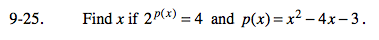Home > A2C > Chapter 9 > Lesson 9.1.1 > Problem9-25

9-25.

Find x if 2p(x) = 4 and p(x) = x2 − 4x − 3. Homework Help ✎Start by finding a common base, then substitute p(x) into the first equation and solve.

x = −1, x = 5